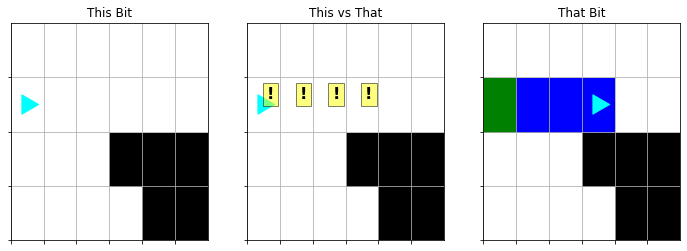Computer Science

# Control Flow

## Legos and Language

When you’re building with Legos, each individual brick is not terribly useful on its own, but once we have enough bricks of different types, we can build some pretty cool stuff.

When you’re learning a new language, sometimes you run into situations where you struggle to express yourself because you don’t know enough words. But as your vocabulary and mastery of the grammar grow, your ability to express yourself becomes more fluent and comfortable.

Today we’ll be presenting more Lego bricks and vocabulary. Each idea by itself is simple, but as you learn to put them together, you’ll do some pretty cool stuff.

## Do you remember…Go Green

``````from byubit import Bit

bit = Bit.new_world(10,3)
while bit.front_clear():
bit.move()
bit.paint("green")
bit.draw()``````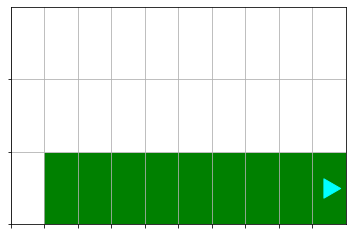The `while` body looks like this:

``````bit.move()
bit.paint("green")``````

That works fine. It paints all the squares but the first one.

We could also have written it this way:

``````bit.paint("green")
bit.move()``````
``````bit = Bit.new_world(10,3)
while bit.front_clear():
bit.paint("green")
bit.move()
bit.draw()``````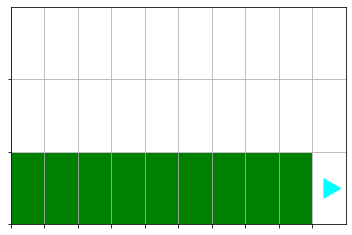That works fine too!

It paints all the squares except the last one.

Loops will very often miss one square - you can decide whether you want to miss the first square or the last square.

This is OK. Don’t go crazy trying to get all the squares in one loop. Just add a line before or after the loop.

``````bit = Bit.new_world(10,3)

# Paint the current square
bit.paint("green")

# Paint the rest
while bit.front_clear():
bit.move()
bit.paint("green")
bit.draw()``````## Looping Intuition

The decision and the action.

Does this make sense to everyone?

## Lines before, in, and after a loop

``````bit = Bit.new_world(10,3)

# Paint the current square
bit.paint("green")

# Paint the rest
while bit.front_clear():
bit.move()
bit.paint("green")
bit.draw()``````

There are lines before the loop. They run once.

The lines in the loop run many (or no) times. It’s flexible.

The lines after the loop run once.

How does the computer know which lines belong to the loop and which lines don’t?

``````bit = Bit.new_world(10,3)

# Paint the current square
bit.paint("green")

# Paint the rest
while bit.front_clear():
bit.move()
bit.paint("green")

bit.paint("red")
bit.draw()``````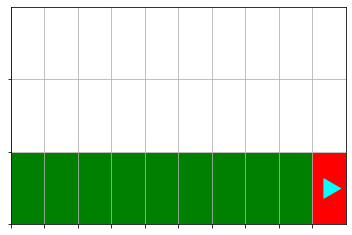What happens if we indent `bit.paint("red")`?

## No Go

``````bit = Bit.new_world(1,1)  # Not a very big space to live in...
while bit.front_clear():
bit.move()
bit.paint("green")

bit.draw()``````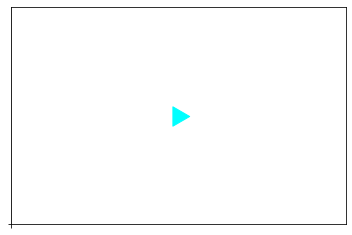How many times does the loop body run?

``````bit = Bit.new_world(5,3)
while bit.front_clear():
bit.move()
bit.paint("green")

bit.draw()``````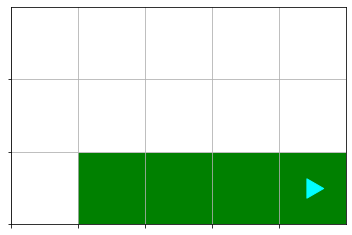How manuy times does the loop body run?

Same loop code, different scenarios -> Generality

## Generality

When you pull into the gas station, you have to find the pump specific for your car’s make and model.

🚗

When you bake a pie, you have to put the pie in the pie-baking oven, not the break-baking oven.

🥧

NO!

Generality is the concept that I can build one thing that will work for many use-cases.

It doesn’t have to work for ALL use-cases, but it probably isn’t useful if it only works in one case.

Usually, we like generalized code. One script works for many scenarios.

When you try to make your code cover too many scenarios…that’s silly.

Usually you’ll have a good feel for the kinds of cases you are trying to solve at the same time, and which cases need a new solution. Like gas pump vs EV charging station.

## `while True`

Recall that a `while` loop needs an expression that evaluates to `True` or `False`.

If the condition is `True`, then the loop body will run.

If the condition is `False`, the loop body won’t run.

After each execution of the loop, we test the condition again.

What happens if the condition never changes?

``````bit = Bit.new_world(3,3)
while True:
bit.draw()
bit.move()
bit.paint("green")
bit.draw()``````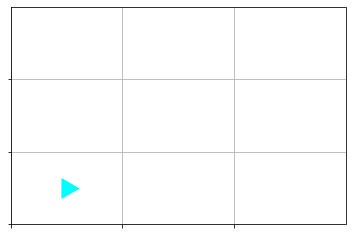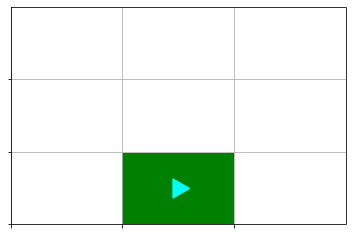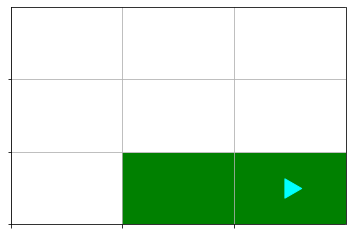``````    ---------------------------------------------------------------------------

MoveOutOfBoundsException                  Traceback (most recent call last)

/tmp/ipykernel_849/3921399024.py in <module>
2 while True:
3     bit.draw()
----> 4     bit.move()
5     bit.paint("green")
6 bit.draw()

/data/teach/cs110/lectures/Lecture2-Bit-control-flow/byubit.py in move(self)
178         next_pos = self._get_next_pos()
179         if not self._pos_in_bounds(next_pos):
--> 180             raise MoveOutOfBoundsException(f"Bit tried to move to {next_pos}, but that is out of bounds")
181         elif self._get_color_at(next_pos) == BLACK:
182             raise MoveBlockedByBlackException(next_pos)

MoveOutOfBoundsException: Bit tried to move to [3 0], but that is out of bounds``````

## Spin!

``````bit = Bit.new_world(3,3)
while True:
bit.left()``````
``````    ---------------------------------------------------------------------------

Exception                                 Traceback (most recent call last)

/tmp/ipykernel_849/2297832775.py in <module>
1 bit = Bit.new_world(3,3)
2 while True:
----> 3     bit.left()

/data/teach/cs110/lectures/Lecture2-Bit-control-flow/byubit.py in left(self)
188         """Turn the bit to the left"""
189         self.orientation = self._next_orientation(1)
--> 190         self._step()
191
192     def right(self):

/data/teach/cs110/lectures/Lecture2-Bit-control-flow/byubit.py in _step(self)
116         self._step_count += 1
117         if self._step_count > MAX_STEP_COUNT:
--> 118             raise Exception("Bit has done too many things. Is he stuck in an infinite loop?")
119
120     def save(self, filename: str):

Exception: Bit has done too many things. Is he stuck in an infinite loop?``````

## A Note on Calling Functions

Python requires `()` for a function call to happen. But it is technically valid syntax to leave the `()` off, but the meaning becomes different.

### `bit.move()`

You: “Hey computer, move the `bit`.”

Computer: “OK”

### `bit.move`

You: “Hey computer, did you know `bit` has an action called `move`?”

Computer: “Yes. I was aware of that.”

``````bit = Bit.new_world(5,3)
while bit.front_clear():
bit.move
bit.paint('blue')``````
``````    ---------------------------------------------------------------------------

Exception                                 Traceback (most recent call last)

/tmp/ipykernel_849/3648138265.py in <module>
1 bit = Bit.new_world(5,3)
----> 2 while bit.front_clear():
3         bit.move
4         bit.paint('blue')

/data/teach/cs110/lectures/Lecture2-Bit-control-flow/byubit.py in front_clear(self)
208         Black squares are not clear.
209         """
--> 210         self._step()
211         return self._space_is_clear(self._get_next_pos())
212

/data/teach/cs110/lectures/Lecture2-Bit-control-flow/byubit.py in _step(self)
116         self._step_count += 1
117         if self._step_count > MAX_STEP_COUNT:
--> 118             raise Exception("Bit has done too many things. Is he stuck in an infinite loop?")
119
120     def save(self, filename: str):

Exception: Bit has done too many things. Is he stuck in an infinite loop?``````

## `if`

`while` loops are great when you want to do the same thing repeatedly.

What if you want to control whether it happens only once?

What if you want to paint a square green if it is blue, but you want to paint it blue if it is something else?

``````bit = Bit.load("blue-to-green.txt")
bit.draw()``````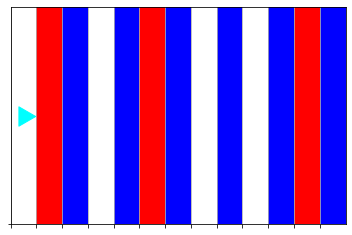``````bit = Bit.load("blue-to-green.txt")
bit.draw()

# Paint green if blue, paint blue for anything else
while bit.front_clear():
bit.move()
if bit.get_color() == "blue":
bit.paint("green")
else:
bit.paint("blue")
bit.draw()``````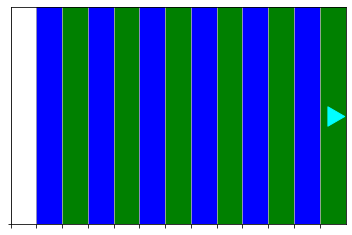👏🏽

That was cool. Let’s dig in.

## `if` syntax

``````if <condition>:
Do this if <condition> is True
else:
Do this if <condition> is False``````

Also valid:

``````if <condition>:
Do this if <condition> is True``````

Again, indentation matters.

`bit.get_color()` returns the color of the square `bit` is currently sitting on.

``````bit = bit.new_world(1,1)
print(bit.get_color())

bit.paint("blue")
print(bit.get_color())

bit.erase()
print(bit.get_color())``````

None blue None

`None` has a special meaning in python. It’s a way of saying “nothing”. You’ll see it used more as we go along.

## Revisiting expressions

``print(2)``

2

``print(1 + 1)``

2

``print(1 + 2 + 3 - 4)``

2

In these expressions, I’m essentially telling the computer:

“Take these symbols, do the work they represent, and bring the result back to me.”

``print(1 + 2 + 3 - 4)``

becomes

``print(2)``

In python, the name of the function (e.g. `bit.get_color`) represents some series of work, just like `+` represents the work of addition.

When you call a function (e.g. `bit.get_color()`), you’re telling the computer:

“Do the work that `bit.get_color` represents, then bring the result back to me.”

When a function has nothing to return, it returns `None`.

``print(bit.left())``

None

So, what does `print` return? How could we find out?

## Comparing values

``````while bit.front_clear():
bit.move()
if bit.get_color() == "blue":
bit.paint("green")
else:
bit.paint("blue")``````

When we want to compare two values—i.e. we want to ask “are these the same?”—we use `==`.

Yes, there are two `=` in `==`.

``print("blue" == "blue")``

True

``print("blue" == "red")``

False

``print(None == "blue")``

False

Use `!=`

``print("blue" != "red")``

True

``print("blue" != "blue")``

False

## `==` and `!=` in action!

``````if bit.get_color() == "green":
# Run this if the current color is green
...

if bit.get_color() == None:
# Run this if there is no color at the current square

...

if bit.get_color() != "red":
# Run this if the color isn't red (i.e. the color is None, "green", or "blue")``````

## Our Bag of Bricks

We now have a growing bag of Lego bricks

• `move`, `left`, `right`
• `paint`
• `front_clear`
• `get_color`
• `==`
• `while`
• `if`

Let’s put these together to build something great.

## Fix the tree

``````bit = Bit.load("tree-before.txt")
bit.draw()``````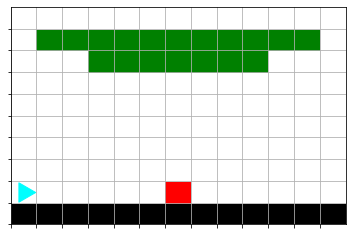``````bit = Bit.load("tree-after.txt")
bit.draw()``````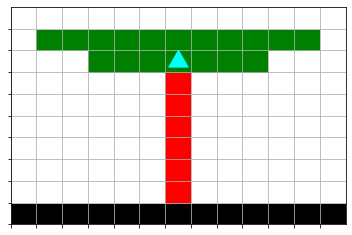When solving problems like these, don’t try to do it all in your head!

Draw pictures.

Write it out.

Let’s break the process down.### What is the next goal—what does that look like?### What code can get Bit from the start to the first goal?

Q: What do we want to do?

A: Move Bit from the start to the red square.

“Move Bit until the square is red”

OR

“While the current square is not red, move the Bit”

How do we know what color the square is?

`bit.get_color()`

How do we know whether that color is not red?

`bit.get_color() != "red"`

How do we move the bit while the color is not red?

``````while bit.get_color() != "red":
bit.move()``````

### Let’s try what we have so far

``````from byubit import Bit
bit.draw()

# Fill it in

bit.draw()``````
GO TEAM! 💪🏻

### What’s the next goal?

We just moved to the red square.

Now we want to move to the green square, but paint as we go.

``````bit = Bit.load("tree-before.txt")
bit.draw()

# Move to the red square
while bit.get_color() != "red":
bit.move()
bit.draw()

# Move up to the green, painting red as we go

big.draw()``````

### Oops!

What happened?

``bit.draw()``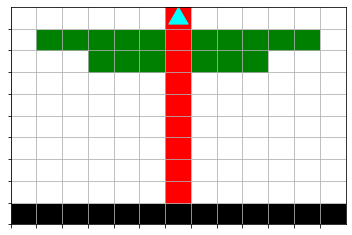That’s not quite what we expected.

Rather than ask: “Why didn’t this work”, let’s ask “What sequence of steps did Bit take to get here”?

Let’s look at the code:

``````# Move to the green square and paint
bit.left()
while bit.get_color() != 'green':
bit.move()
bit.paint('red')
bit.draw()``````

How does the paint red and `while` condition interact?

• Am I on a green color?
• No, I’m on a red color, so move and paint red
• Am I on a green color?
• No, I’m on a red color, so move and paint red
• Am I on a green color?
• No, I’m on a red color, so move and paint red
• Am I on a green color?
• No, I’m on a red color, so move and paint red
🤔

### Solution

So, we’re cobbering the color of the square with red before we check whether it is green.

How do we fix it?

There are several ways we could address it.

Let’s say we only want to paint empty squares red. Then we’ll never clobber a colored square.

``````# Solution - code live
bit.draw()

# Move to the red square
while bit.get_color() != "red":
bit.move()
bit.draw()

# Move to the green square and paint
bit.left()
while bit.get_color() != 'green':
bit.move()
if bit.get_color() == None:
bit.paint('red')
bit.draw()``````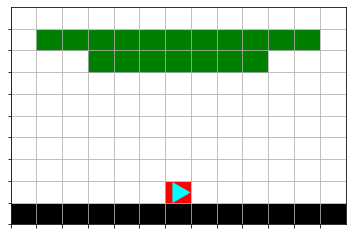``````bit = Bit.load("tree-before.txt")
bit.draw()

# Move to the red square
while bit.get_color() != "red":
bit.move()
bit.draw()

# Move to the green square and paint
# Something isn't working right...please fix it.
bit.left()
while bit.get_color() != 'green':
bit.move()
bit.paint('red')
bit.draw()``````

#### Other solution

We could add a `bit.move()` before our second `while` block and then switch the order of `bit.move()` and `bit.paint()` in the `while` block.

``````bit = Bit.load("tree-before.txt")
bit.draw()

# Move to the red square
while bit.get_color() != "red":
bit.move()
bit.draw()

# Move to the green square and paint
# Something isn't working right...please fix it.
bit.left()
while bit.get_color() != 'green':
bit.move()
bit.paint('red')
bit.draw()``````

Either solution is good.

Each person will have an individual preference for which solution they prefer.

That’s OK!

## Thinking, Drawing, Coding

This isn’t a “beginner’s crutch”—seasoned professionals do this too!

## More bricks for the bag

``````bit.left_clear()
bit.right_clear()``````

These are like `bit.front_clear()`, but checks on the side.

### Blocked squares

Sometimes Bit will encounter blocked squares. They are colored black.

``````bit = Bit.load("blocked-squares.txt")
bit.draw()

print("Front clear: ")
print(bit.front_clear())

print("Left clear: ")
print(bit.left_clear())

print("Right clear: ")
print(bit.right_clear())``````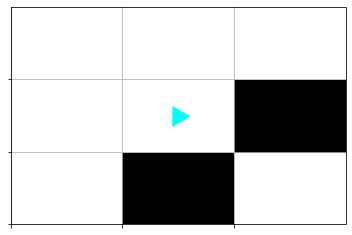Front clear: False Left clear: True Right clear: False

``````bit = Bit.load('while-right-clear.txt')
bit.draw()

while bit.right_clear():
bit.move()

bit.draw()``````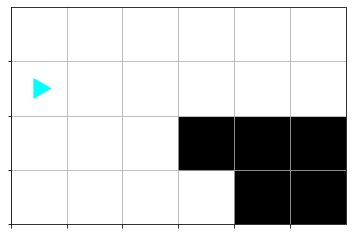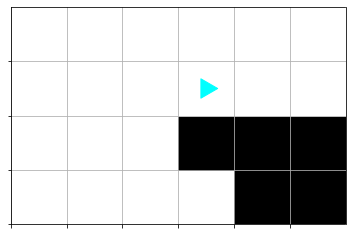### `not`

`not` in python changes a `True` to `False` and a `False` to `True`.

``````while not bit.right_clear():
bit.move()``````
``print(not True)``

False

## Bit Puzzles

https://byucs110.org/resources/bit-puzzles/

## Reverse Coyote

Remember Wiley Coyote from the old cartoon Roadrunner?

He’s always running off of cliffs and suspends in the air for a moment before falling.

Sometimes he’s able to run back to the cliff before he falls. Let’s help him out.

``````bit = Bit.load("reverse-coyote-start.txt")
bit.draw()````````Bit.load("reverse-coyote-finish.txt").draw()``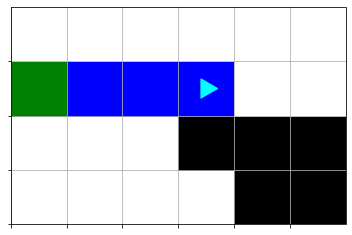What is the test for this? Under what conditions do we want Bit to move?

``````bit = Bit.load("reverse-coyote-start.txt")

# fill in the rest!

``````    Color at 0,2 does not match: None vs green